## 5.2 Assignment Statements

(1)
An assignment_statement replaces the current value of a variable with the result of evaluating an expression.
##### Syntax
```(2)
assignment_statement ::=
variable_name := expression;
```
(3)
The execution of an assignment_statement includes the evaluation of the expression and the assignment of the value of the expression into the target. An assignment operation (as opposed to an assignment_statement) is performed in other contexts as well, including object initialization and by-copy parameter passing. The target of an assignment operation is the view of the object to which a value is being assigned; the target of an assignment_statement is the variable denoted by the variable_name.
##### Name Resolution Rules
(4)
The variable_name of an assignment_statement is expected to be of any nonlimited type. The expected type for the expression is the type of the target.
##### Legality Rules
(5)
The target denoted by the variable_name shall be a variable.
(6)
If the target is of a tagged class-wide type T'Class, then the expression shall either be dynamically tagged, or of type T and tag-indeterminate (see 3.9.2).
##### Dynamic Semantics
(7)
For the execution of an assignment_statement, the variable_name and the expression are first evaluated in an arbitrary order.
(8)
When the type of the target is class-wide:
(9)
• If the expression is tag-indeterminate (see 3.9.2), then the controlling tag value for the expression is the tag of the target;
(10)
• Otherwise (the expression is dynamically tagged), a check is made that the tag of the value of the expression is the same as that of the target; if this check fails, Constraint_Error is raised.
(11)
The value of the expression is converted to the subtype of the target. The conversion might raise an exception (see 4.6).
(12)
In cases involving controlled types, the target is finalized, and an anonymous object might be used as an intermediate in the assignment, as described in 7.6.1, ``Completion and Finalization''. In any case, the converted value of the expression is then assigned to the target, which consists of the following two steps:
(13)
• The value of the target becomes the converted value.
(14)
• If any part of the target is controlled, its value is adjusted as explained in clause 7.6.

NOTES
(15)
(2) The tag of an object never changes; in particular, an assignment_statement does not change the tag of the target.
(16)
(3) The values of the discriminants of an object designated by an access value cannot be changed (not even by assigning a complete value to the object itself) since such objects are always constrained; however, subcomponents of such objects may be unconstrained.
##### Examples
(17)
Examples of assignment statements:
```(18)
Value := Max_Value - 1;
(19)
Next_Frame(F)(M, N) := 2.5;        --  see 4.1.1
U := Dot_Product(V, W);            --  see 6.3
(20)
Writer := (Status => Open, Unit => Printer, Line_Count => 60);  -- see 3.8.1
Next_Car.all := (72074, null);    --  see 3.10.1
```
(21)
Examples involving scalar subtype conversions:
```(22)
I, J : Integer range 1 .. 10 := 5;
K    : Integer range 1 .. 20 := 15;
...
(23)
I := J;  --  identical ranges
K := J;  --  compatible ranges
J := K;  --  will raise Constraint_Error if K > 10
```
(24)
Examples involving array subtype conversions:
```(25)
A : String(1 .. 31);
B : String(3 .. 33);
...
(26)
A := B;  --  same number of components
(27)
A(1 .. 9)  := "tar sauce";
A(4 .. 12) := A(1 .. 9);  --  A(1 .. 12) = "tartar sauce"
```

NOTES
(28)
(4) Notes on the examples: Assignment_statements are allowed even in the case of overlapping slices of the same array, because the variable_name and expression are both evaluated before copying the value into the variable. In the above example, an implementation yielding A(1 .. 12) = "tartartartar" would be incorrect.

####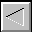|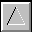|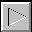|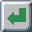|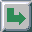TOC -- / --.-- / --.--.-- | Index | Search | Syntax | Help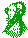-- Email comments, additions, corrections, gripes, kudos, etc. to:

Magnus Kempe -- Magnus.Kempe@di.epfl.ch